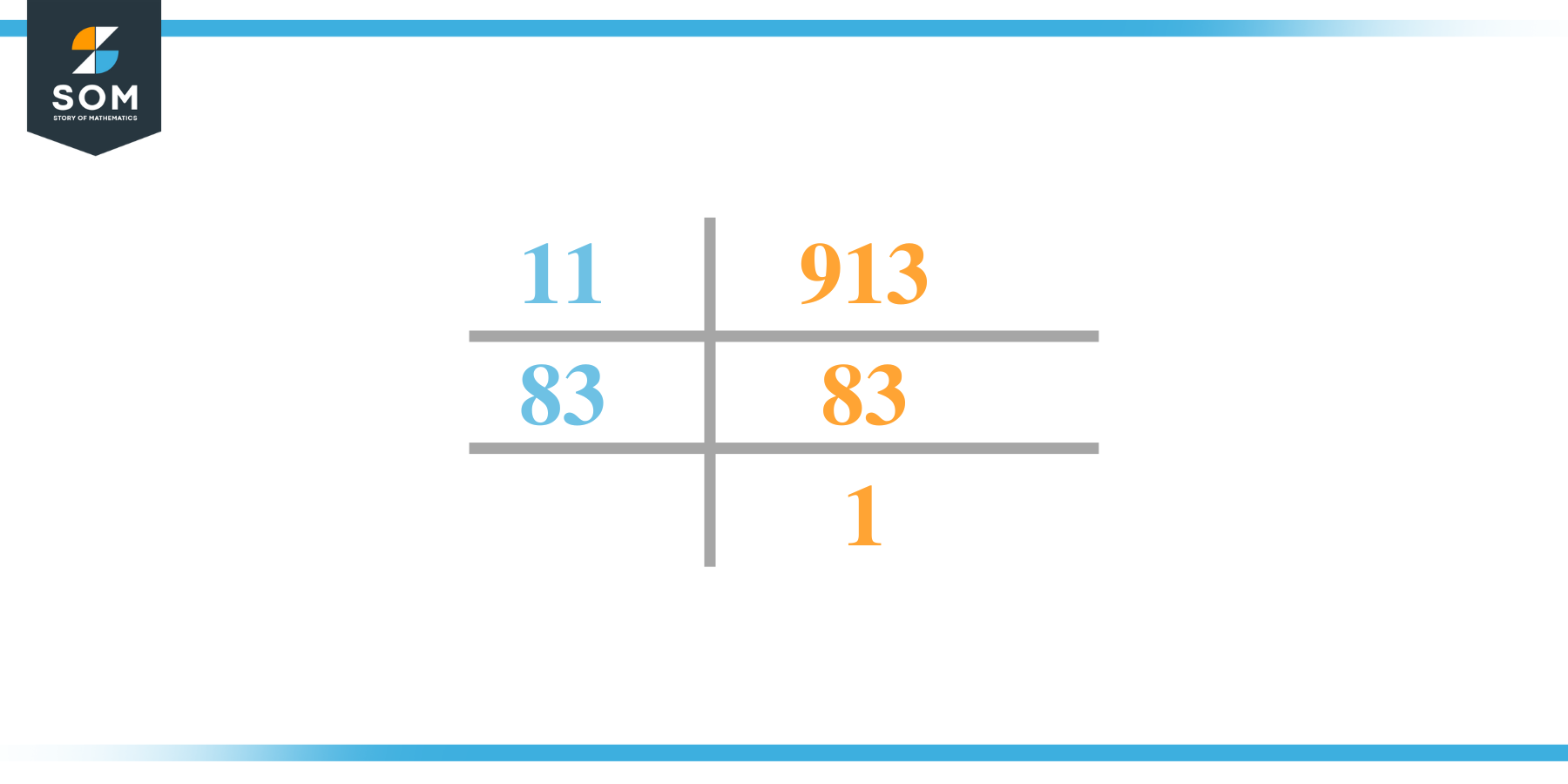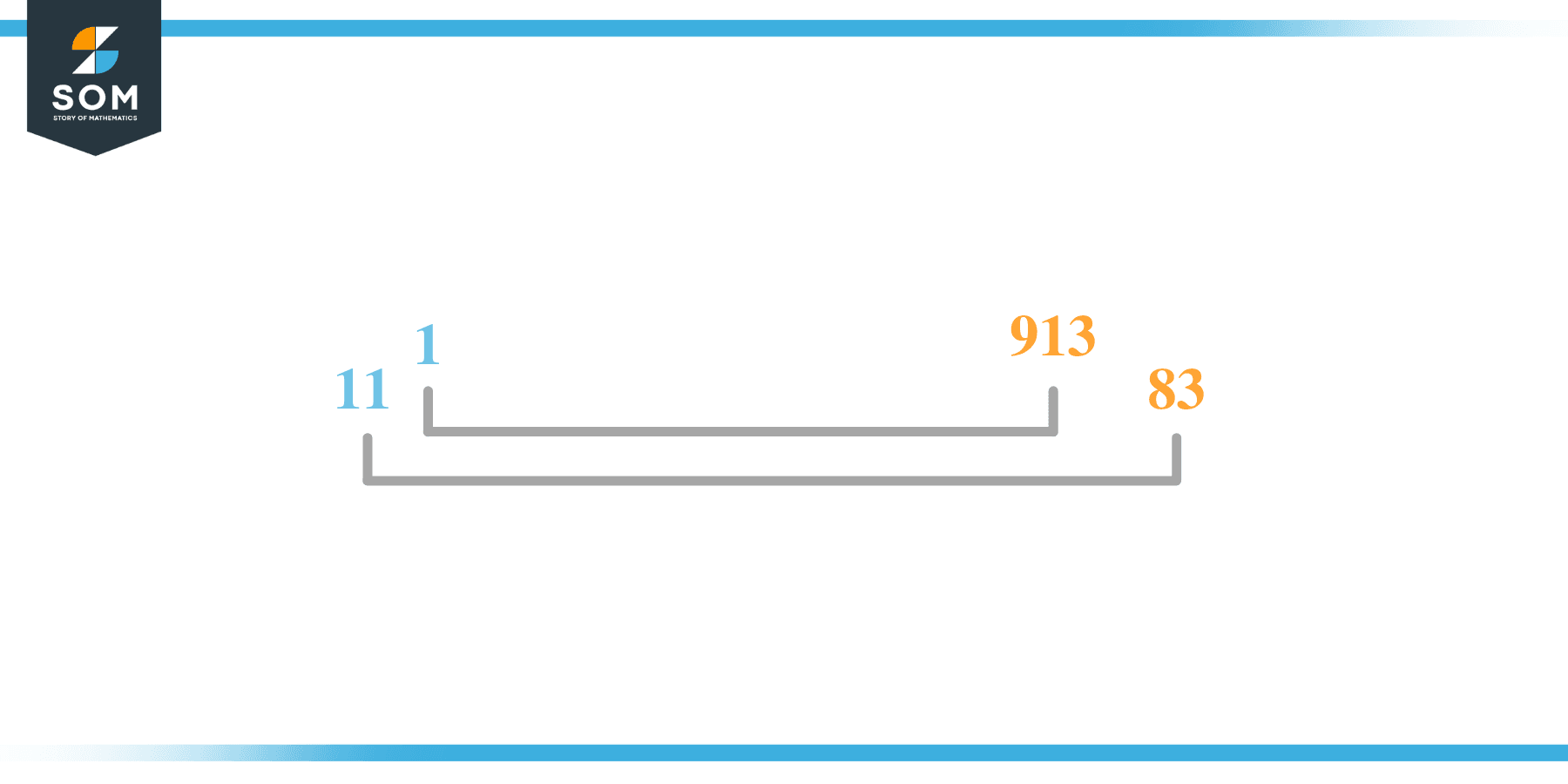# Factors of 913: Prime Factorization, Methods, and Examples

The numbers that may divide 913 completely are considered to be its factors. With just two elements, the number 913 is composite. Positive and negative factors are both possible.We will study the components of 913 in this session.

### Factors of 913

Here are the factors of number 913.

Factors of 913: 1, 11, 83, and 913

### Negative Factors of 913

The negative factors of 913 are similar to its positive aspects, just with a negative sign.

Negative Factors of 913: -1, -11, -83, and -913

### Prime Factorization of 913

The prime factorization of 913 is the way of expressing its prime factors in the product form.

Prime Factorization:11 x 83

In this article, we will learn about the factors of 913 and how to find them using various techniques such as upside-down division, prime factorization, and factor tree.

## What Are the Factors of 913?

The factors of 913 are 1, 11, 83, and 913. These numbers are the factors as they do not leave any remainder when divided by 913.

The factors of 913 are classified as prime numbers and composite numbers. The prime factors of the number 913 can be determined using the prime factorization technique.

## How To Find the Factors of 913?

You can find the factors of 913 by using the rules of divisibility. The divisibility rule states that any number, when divided by any other natural number, is said to be divisible by the number if the quotient is the whole number and the resulting remainder is zero.

To find the factors of 913, create a list containing the numbers that are exactly divisible by 913 with zero remainders. One important thing to note is that 1 and 913  are the 913’s factors as every natural number has 1 and the number itself as its factor.

1 is also called the universal factor of every number. The factors of 913 are determined as follows:

$\dfrac{913}{1} = 913$

$\dfrac{913}{11} = 83$

$\dfrac{913}{83} = 11$

$\dfrac{913}{913} = 1$

Therefore, 1, 11, 83, and 913are the factors of 913.

### Total Number of Factors of 913

For 913, there are 4 positive factors and 4 negative ones. So in total, there are 8 factors out of 913.

To find the total number of factors of the given number, follow the procedure mentioned below:

1. Find the factorization/prime factorization of the given number.
2. Demonstrate the prime factorization of the number in the form of exponent form.
3. Add 1 to each of the exponents of the prime factor.
4. Now, multiply the resulting exponents together. This obtained product is equivalent to the total number of factors of the given number.

By following this procedure, the total number of factors of 913 is given as:

Factorization of 913 is 1 x 11 x 83.

The exponent of 1 is 1, 11 is 1, and 83 is 1.

Adding 1 to each and multiplying them together results in 8.

Therefore, the total number of factors 913 is 8. 4 are positive, and 4 factors are negative.

### Important Notes

Here are some essential points that must be considered while finding the factors of any given number:

• The factor of any given number must be a whole number.
• The factors of the number cannot be in the form of decimals or fractions.
• Factors can be positive as well as negative.
• Negative factors are the additive inverse of the positive factors of a given number.
• The factor of a number cannot be greater than that number.
• Every even number has 2 as its prime factor, the smallest prime factor.

## Factors of 913 by Prime FactorizationThe number 913 is a composite/prime number. Prime factorization is a valuable technique for finding the number’s prime factors and expressing the number as the product of its prime factors.

Before finding the factors of 913 using prime factorization, let us find out what prime factors are. Prime factors are the factors of any given number that are only divisible by 1 and themselves.

To start the prime factorization of 913, start dividing by its most minor prime factor. First, determine that the given number is either even or odd. If it is an even number, then 2 will be the smallest prime factor.

Continue splitting the quotient obtained until 1 is received as the quotient. The prime factorization of 913 can be expressed as:

913 = 11 x 83

## Factors of 913 in PairsThe factor pairs are the duplet of numbers that, when multiplied together, result in the factorized number. Factor pairs can be more than one depending on the total number of factors given.

For 913, the factor pairs can be found as:

1 x 913 = 913

11 x 83 = 913

The possible factor pairs of 913 are given as (1, 913) and (11, 83 ).

All these numbers in pairs, when multiplied, give 913 as the product.

The negative factor pairs of 913 are given as:

-1 x -913 = 913

-11 x -83 = 913

It is important to note that in negative factor pairs, the minus sign has been multiplied by the minus sign, due to which the resulting product is the original positive number. Therefore, -1, -11, -83, and -913 are called negative factors of 913.

The list of all the factors of 913, including positive as well as negative numbers, is given below.

Factor list of 913: 1,-1, 11,-11, 83,-83,  913, and -913

## Factors of 913 Solved Examples

To better understand the concept of factors, let’s solve some examples.

### Example 1

How many factors of 913 are there?

### Solution

The total number of Factors of 913 is 8.

Factors of 913 are 1, 11, 83, and 913.

### Example 2

Find the factors of 913 using prime factorization.

### Solution

The prime factorization of 913 is given as:

913 $\div$ 11 = 83

83 $\div$ 83 = 1

So the prime factorization of 913 can be written as:

11 x 83 = 913